lambertw

Lambert W function

Description

example

lambertw(x) returns the principal branch of the Lambert W function. This syntax is equivalent to lambertw(0,x).

example

lambertw(k,x) is the kth branch of the Lambert W function. This syntax returns real values only if k = 0 or k = -1.

Examples

collapse all

The Lambert W function W(x) is a set of solutions of the equation x = W(x)eW(x).

Solve this equation. The solution is the Lambert W function.

syms x W
eqn = x == W*exp(W);
solve(eqn,W)
ans =
lambertw(0, x)

Verify that branches of the Lambert W function are valid solutions of the equation x = W*eW:

k = -2:2;
eqn = subs(eqn,W,lambertw(k,x));
isAlways(eqn)
ans =
1×5 logical array
1     1     1     1     1

Depending on its arguments, lambertw can return floating-point or exact symbolic results.

Compute the Lambert W functions for these numbers. Because the numbers are not symbolic objects, you get floating-point results.

A = [0 -1/exp(1); pi i];
lambertw(A)
ans =
0.0000 + 0.0000i  -1.0000 + 0.0000i
1.0737 + 0.0000i   0.3747 + 0.5764i
lambertw(-1,A)
ans =
-Inf + 0.0000i  -1.0000 + 0.0000i
-0.3910 - 4.6281i  -1.0896 - 2.7664i

Compute the Lambert W functions for the numbers converted to symbolic objects. For most symbolic (exact) numbers, lambertw returns unresolved symbolic calls.

A = [0 -1/exp(sym(1)); pi i];
W0 = lambertw(A)
W0 =
[               0,             -1]
[ lambertw(0, pi), lambertw(0, 1i)]
Wmin1 = lambertw(-1,A)
Wmin1 =
[             -Inf,              -1]
[ lambertw(-1, pi), lambertw(-1, 1i)]

Convert symbolic results to double by using double.

double(W0)
ans =
0.0000 + 0.0000i  -1.0000 + 0.0000i
1.0737 + 0.0000i   0.3747 + 0.5764i

Plot the two main branches, ${W}_{0}\left(x\right)$ and ${W}_{-1}\left(x\right)$, of the Lambert W function.

syms x
fplot(lambertw(x))
hold on
fplot(lambertw(-1,x))
hold off
axis([-0.5 4 -4 2])
title('Lambert W function, two main branches')
legend('k=0','k=1','Location','best')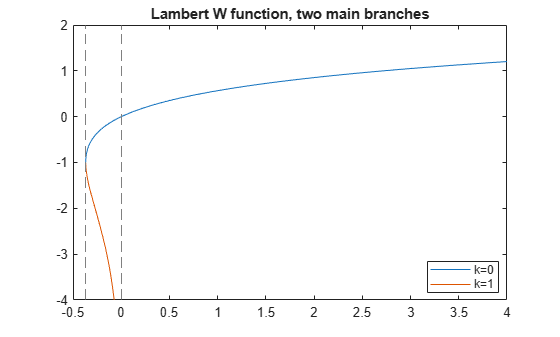Plot the principal branch of the Lambert W function on the complex plane.

Plot the real value of the Lambert W function by using fmesh. Simultaneously plot the contours by setting 'ShowContours' to 'On'.

syms x y
f = lambertw(x + 1i*y);
interval = [-100 100 -100 100];
fmesh(real(f),interval,'ShowContours','On')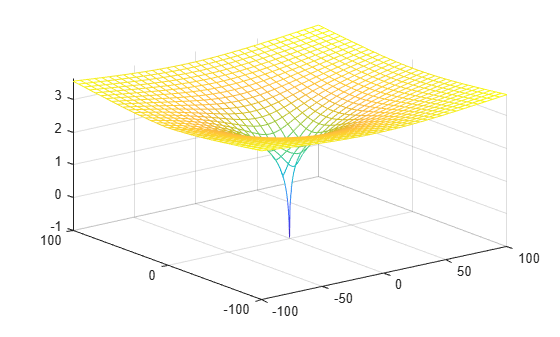Plot the imaginary value of the Lambert W function. The plot has a branch cut along the negative real axis. Plot the contours separately.

fmesh(imag(f),interval)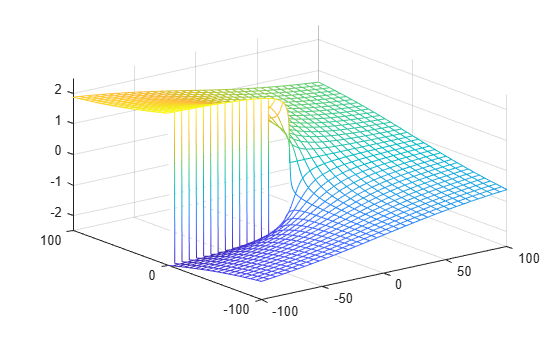fcontour(imag(f),interval,'Fill','on')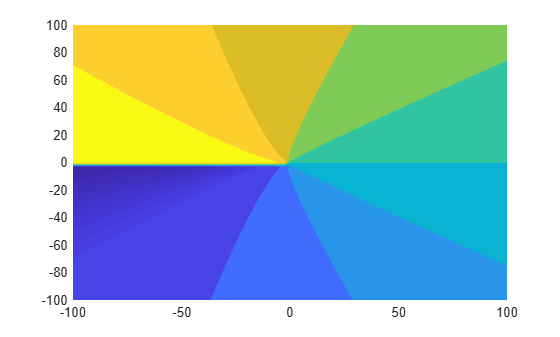Plot the absolute value of the Lambert W function.

fmesh(abs(f),interval,'ShowContours','On')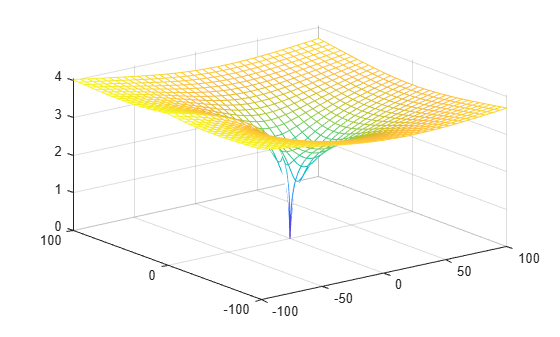Input Arguments

collapse all

Input, specified as a number, vector, matrix, or array, or a symbolic number, variable, array, function, or expression.

At least one input argument must be a scalar, or both arguments must be vectors or matrices of the same size. If one input argument is a scalar and the other is a vector or matrix, lambertw expands the scalar into a vector or matrix of the same size as the other argument with all elements equal to that scalar.

Branch of Lambert W function, specified as an integer, a vector or matrix of integers, a symbolic integer, or a symbolic vector or matrix of integers.

At least one input argument must be a scalar, or both arguments must be vectors or matrices of the same size. If one input argument is a scalar and the other is a vector or matrix, lambertw expands the scalar into a vector or matrix of the same size as the other argument with all elements equal to that scalar.

collapse all

Lambert W Function

The Lambert W function W(x) represents the solutions y of the equation $y{e}^{y}=x$ for any complex number x.

• For complex x, the equation has an infinite number of solutions y = lambertW(k,x) where k ranges over all integers.

• For all real x ≥ 0, the equation has exactly one real solution y = lambertW(x) = lambertW(0,x).

• For real x where $-{e}^{-1}, the equation has exactly two real solutions. The larger solution is represented by y = lambertW(x) and the smaller solution by y = lambertW(–1,x).

• For $x=-{e}^{-1}$, the equation has exactly one real solution y = –1 = lambertW(0, –exp(–1)) = lambertW(–1, -exp(–1)).

 Corless, R.M., G.H. Gonnet, D.E.G. Hare, D.J. Jeffrey, and D.E. Knuth. "On the Lambert W Function." Advances in Computational Mathematics, Vol. 5, pp. 329–359, 1996.

Symbolic Math Toolbox Documentation

Mathematical Modeling with Symbolic Math Toolbox

Get examples and videos